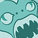# App Development

Announcements
QlikWorld 2023, a live, in-person thrill ride. Save \$300 before February 6: REGISTER NOW!
cancel
Showing results for
Did you mean:Contributor III

## Create period as dimension

Hi,

This is my code in a dimension in straight tab

=if([TOREP_COMPLETED_DATE] >= Monthstart(AddMonths(Today(), -1)) , 'P1M',
if([TOREP_COMPLETED_DATE] >= Monthstart(AddMonths(Today(),-3)) and TOREP_COMPLETED_DATE <= MonthEnd(TOREP_COMPLETED_DATE, -1), 'P3M',
if([TOREP_COMPLETED_DATE] >= Monthstart(AddMonths(Today(),-12)) and TOREP_COMPLETED_DATE <= MonthEnd(TOREP_COMPLETED_DATE, -1), 'P12M',

My Question :

Each condition (if) is evaluated according to the result of the previous one. It's right.However, I wish to evaluate each condition from the beginning and without taking into account the previous result in order to have the right amount for each period.

Fred

1 Solution

Accepted SolutionsMaster

My mistake change in expression

if([TOREP_COMPLETED_DATE] >= Monthstart(AddMonths(Today(), -1)) , 1,0) as 'P1M_Flag',

if([TOREP_COMPLETED_DATE] >= Monthstart(AddMonths(Today(),-3)) and TOREP_COMPLETED_DATE <= MonthEnd(TOREP_COMPLETED_DATE, -1),1,0) as  'P3M_Flag',

if([TOREP_COMPLETED_DATE] >= Monthstart(AddMonths(Today(),-12)) and TOREP_COMPLETED_DATE <= MonthEnd(TOREP_COMPLETED_DATE, -1),1,0) as  'P12M_Flag',

if([TOREP_COMPLETED_DATE] >= YearStart(AddYears(Today(), 0)) and [TOREP_COMPLETED_DATE] <= Monthstart(AddMonths(Today(), -1)), 1,0) As 'YTD_Flag'

.

.

etc

From Table ;

DIM: ValueList('P1M','P3M','P12M','YTD')

Expression: If(ValueList('P1M','P3M','P12M','YTD') ='P1M', Sum({<P1M_Flag={'1'}>}Sales),

if(ValueList('P1M','P3M','P12M','YTD') ='P3M',Sum({<P3M_Flag={'1'}>}Sales),

if(ValueList('P1M','P3M','P12M','YTD') ='P12M',Sum({<P12M_Flag={'1'}>}Sales),

if(ValueList('P1M','P3M','P12M','YTD') ='YTD',Sum({<YTD_Flag={'1'}>}Sales),

))))

Thanks,
Sasi

5 RepliesMaster

HI,

Not a solution to your problem but its another way to get the required output

Creates flags at script level for all these conditions and use them in the chart.

--------------------------------------------------------------------------------------------------------------

if([TOREP_COMPLETED_DATE] >= Monthstart(AddMonths(Today(), -1)) , 1,0) as 'P1M',

if([TOREP_COMPLETED_DATE] >= Monthstart(AddMonths(Today(),-3)) and TOREP_COMPLETED_DATE <= MonthEnd(TOREP_COMPLETED_DATE, -1),1,0) as  'P3M',

.

.

etc

From Table ;

----------------------------------------------------------------------------------------------------------------------

Create a synthetic/Calculated dimension (Value List)

DIM: ValueList('P1M','P3M','P12M','YTD')

Expression: If(ValueList('P1M','P3M','P12M','YTD') ='P1M', Sum(Sales),

if(ValueList('P1M','P3M','P12M','YTD') ='P3M',sum(Sales),

if(ValueList('P1M','P3M','P12M','YTD') ='P12M',sum(Sales),

if(ValueList('P1M','P3M','P12M','YTD') ='YTD',sum(Sales)

))))

Thanks,
SasiContributor III
Author

Hi Sasi,

Thanks for this solution.

After created my Tab with the Valuelist and flag on script, all result of each expression are the same result and any difference between flag.

I not understand this case. Can you help me again ?

FredMaster

My mistake change in expression

if([TOREP_COMPLETED_DATE] >= Monthstart(AddMonths(Today(), -1)) , 1,0) as 'P1M_Flag',

if([TOREP_COMPLETED_DATE] >= Monthstart(AddMonths(Today(),-3)) and TOREP_COMPLETED_DATE <= MonthEnd(TOREP_COMPLETED_DATE, -1),1,0) as  'P3M_Flag',

if([TOREP_COMPLETED_DATE] >= Monthstart(AddMonths(Today(),-12)) and TOREP_COMPLETED_DATE <= MonthEnd(TOREP_COMPLETED_DATE, -1),1,0) as  'P12M_Flag',

if([TOREP_COMPLETED_DATE] >= YearStart(AddYears(Today(), 0)) and [TOREP_COMPLETED_DATE] <= Monthstart(AddMonths(Today(), -1)), 1,0) As 'YTD_Flag'

.

.

etc

From Table ;

DIM: ValueList('P1M','P3M','P12M','YTD')

Expression: If(ValueList('P1M','P3M','P12M','YTD') ='P1M', Sum({<P1M_Flag={'1'}>}Sales),

if(ValueList('P1M','P3M','P12M','YTD') ='P3M',Sum({<P3M_Flag={'1'}>}Sales),

if(ValueList('P1M','P3M','P12M','YTD') ='P12M',Sum({<P12M_Flag={'1'}>}Sales),

if(ValueList('P1M','P3M','P12M','YTD') ='YTD',Sum({<YTD_Flag={'1'}>}Sales),

))))

Thanks,
SasiContributor III
Author

Very thanks Sasi for your knowledge ! It works.

Now, I set the end date with today().

I would like the user to be able to choose the end date (reference date).

Example: choose 13 march 2019 -> P1M = February 2019 and P1M-1 = February 2018. etc. for PM3, P3M-1, ..., YTD, YTD-1.

What to change in the script?

thank you very much for your help, I'm not very used to the qlik language yet.

FredContributor III
Author

Sasi,

Very thanks for your knowledge ! It works.

One question :

Now, I set the end date with today().

I would like the user to be able to choose the end date (reference date).

Example: choose 13 march 2019 -> P1M = February 2019 and P1M-1 = February 2018. etc. for PM3, P3M-1, ..., YTD, YTD-1.

What to change in the script?

thank you very much for your help, I'm not very used to the qlik language yet.

FredTags
Community Browser